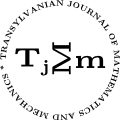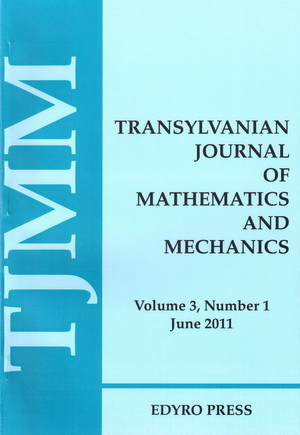ISSN: 2067-239X
ISSN(on-line): 2067-239X

Indexed in:
Mathematical Reviews
Zentralblatt MATH
EBSCO

Front cover# Volume 2 (2010), Number 1

## One Derivative of One Component Regularity Criterion for the Navier-Stokes Equations

### Author(s): YUAN-SHAN ZHAO AND YUE HU

Abstract: We study the incompressible Navier-Stokes equations in the entire three-dimensional space and we prove that if there exists one derivative of one component of the velocity ${\partial }_{3}{u}_{3}\in {L}_{t}^{s}{L}_{x}^{r},\text{\hspace{0.17em}}\text{where}\text{\hspace{0.17em}}\frac{2}{s}+\frac{3}{r}\le \frac{1}{4},\text{\hspace{0.17em}}12\le r\le \infty$ then the solution is regular. This extends one result of Patrick Penel, Toulon, Milan Pokorý, Praha [Appl.Math.,49,483-493(2004)]

## On Some Multiplier Difference Sequence Spaces Defined over a 2-normed Linear Space

### Author(s): B.SURENDER REDDY AND HEMEN DUTTA

Abstract: In this paper, we introduce a new class of generalized difference sequences with base space, a real linear 2-normed space and by means of a fixed multiplier. We study the spaces of thus constructed classes of sequences for relevant linear topological structures. Further we investigate the spaces for solidity, monotonicity, symmetricity etc. We also obtain some relations between these spaces as well as prove some inclusion results.

## On Some Inequalities of Simpson-type Via Quasi-Convex Functions and Applications

### Author(s): MOHAMMAD ALOMARI AND MASLINA DARUS

Abstract: Some inequalities of Simpson's type for quasi-convex functions are introduced. In the literature the error estimates for the midpoint rule is $|{E}_{mid}\left(f,d\right)|\le \frac{K}{24}\sum _{i=0}^{n-1}{\left({x}_{i+1}-{x}_{i}\right)}^{3}$ , in this paper we restrict the conditions on f to get better error estimates than the original.

## Solving Cauchy Problem for a Class of Sixth-order Hyperbolic Equations with Triple Characteristics

### Author(s): LAZHAR BOUGOFFA AND HIND K. AL-JEAID

Abstract: In this paper, the Cauchy problem for a class of the homogeneous hyperbolic equations for sixth-order with triple characteristics is considered and can be solved analytically by direct integration techniques. Also, an efficient modification of Adomian decomposition method is proposed for solving this type of problems. We then conduct a comparative study between the ADM and direct method with the help of several illustrative examples.

## New Explicit and Implicit Solutions to Elliptic Equations with Two Space Variables

Abstract: We present a direct method for finding a new explicit and implicit solutions of elliptic equations with hyperbolic, trigonometric and exponential nonlinearities.

## BAICA-CARDU Paratrigonometry, a Generalization of the Classical and Some New Non-classical Trigonometries and Its Application in Mechanics and Wave Theory

### Author(s): M. BAICA AND M.CARDU

Abstract: In their previous papers the authors introduced some new Trigonometries as: 1. Quadratic Trigonometry (QT), 2. Polygonal Trigonometry (PT), 3. Trans Trigonometry (TT), 4. Infra Trigonometry (IT), 5. Ultra-Trigonometry (UT), 6. Extra Trigonometry (ET), 7. Para-Trigonometry (PRT). This time in this paper we perform a synthesis of all these Trigonometries and state some of their applications.

## Oscillation of a Class of Two-variables Functional Equations with Variable Coefficients

### Author(s): WENGUI YANG

Abstract: In this paper we will establish some sufficient conditions of oscillation of a class of two-variables functional equations with variable coefficients. Our results extend Zhang and Zhou's results (B.G. Zhang and Y. Zhou, Comput. Math. Appl. 42 (3-5) (2001) 369-378).

## On Algebraic Properties of the Generalized Chebyshev Polynomials

### Author(s): AHMET İPEK

Abstract: Chebyshev polynomials are of great importance in many areas of mathematics, particularly approximation theory. Numerous articles and books have been written about this topic. Analytical properties of Chebyshev polynomials are well understood, but algebraic properties less so. In this paper, new generalized Chebyshev polynomials of the first and second kinds have been introduced and studied. Many of the properties of these polynomials are proved.

## A Note on Bounds for the Spectral Norms of Circulant-Cauchy-Toeplitz Matrices

### Author(s): AHMET İPEK

Abstract: In this paper, we established lower and upper bounds for the spectral norms of some Circulant-Cauchy-Toeplitz matrices.

## A Nonlinear Mixed Type Volterra-Fredholm Functional Integral Equation Via Perov's Theorem

Abstract: In this paper we study the following mixed type Volterra-Fredholm functional integral equation $x\left(t\right)=F\left(t,x\left(t\right),{\int }_{{a}_{1}}^{{t}_{1}}\dots {\int }_{{a}_{m}}^{{t}_{m}}K\left(t,s,x\left(s\right)\right)ds,{\int }_{{a}_{1}}^{{b}_{1}}\dots {\int }_{{a}_{m}}^{{b}_{m}}H\left(t,s,x\left(s\right)\right)ds\right)$. Using the Perov's Theorem and the Picard operator technique we establish existence, uniqueness, data dependence and Gronwall results for the solutions.

## The Solution of a System of Nonlinear Integral Equations from Physics

### Author(s): MARIA DOBRIŢOIU

Abstract: Using the Perov's Theorem and the General data dependence Theorem, in this paper, we obtain some conditions concerning the existence and uniqueness of the solution in the Banach space $C\left(\left[a,b\right],{ℝ}^{m}\right)$ and the continuous data dependence of the solution of the following system of nonlinear integral equations from physics: $x\left(t\right)={\int }_{a}^{b}K\left(t,s,x\left(s\right),x\left(a\right),x\left(b\right)\right)ds+f\left(t\right),t\in \left[a,b\right]$. An example is also given here.

## Double Inequalities of Boole's Quadrature Rule

### Author(s): MARIUS HELJIU

Abstract: In this paper double inequalities of Boole's type quadrature rule are given. These inequalities are sharp.